# Inputs output costs

21. Nov 2011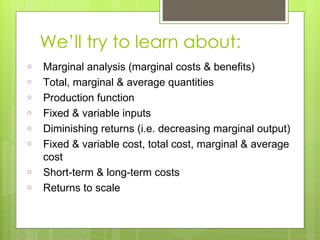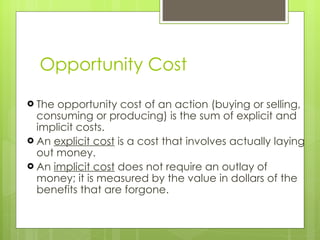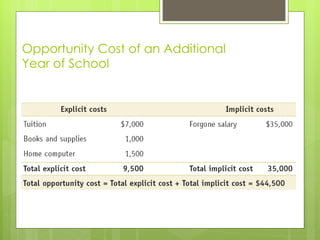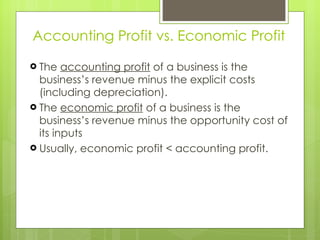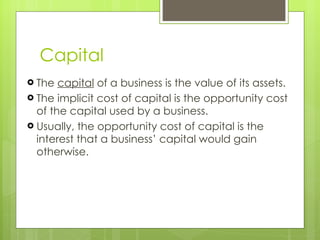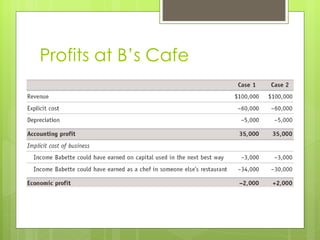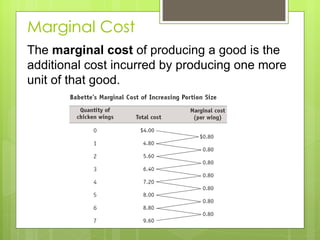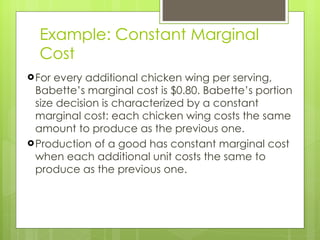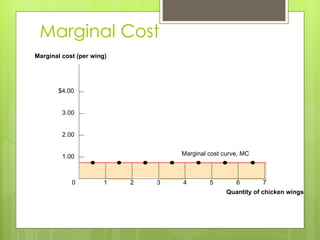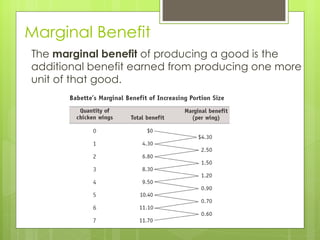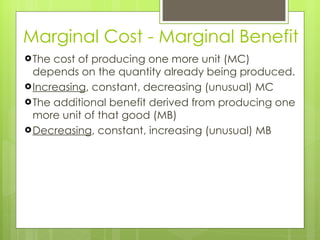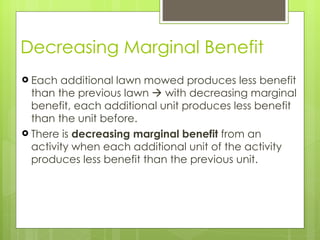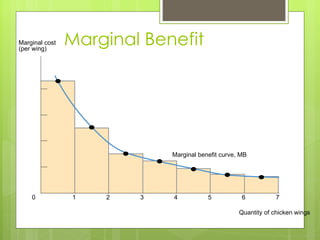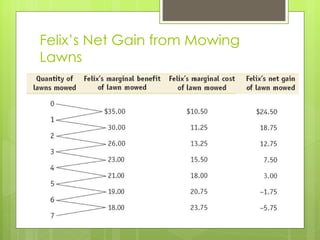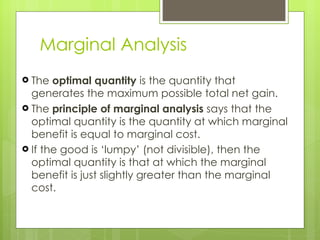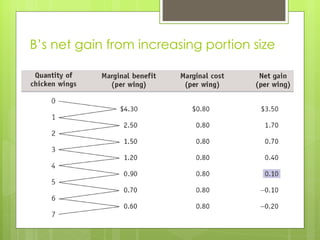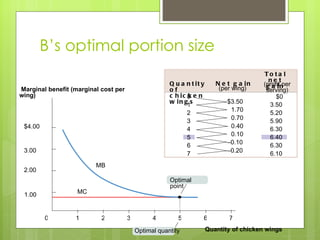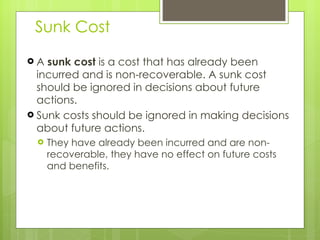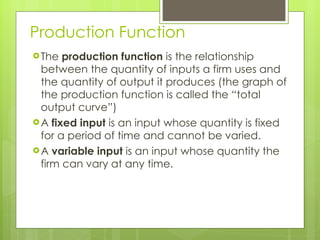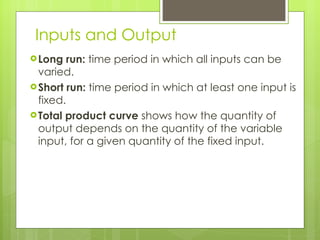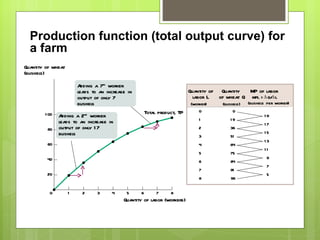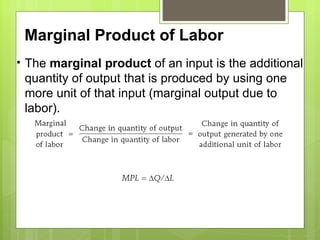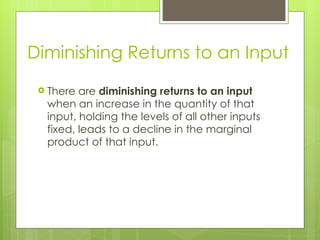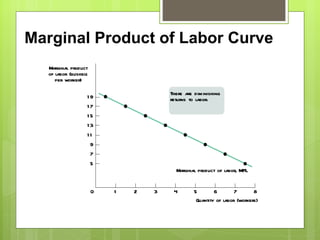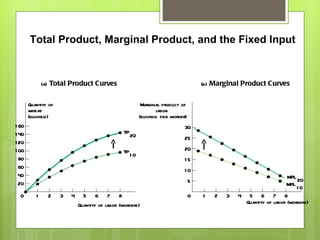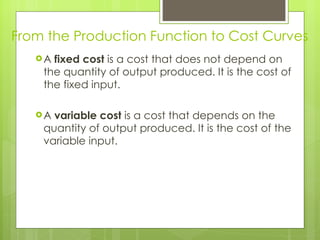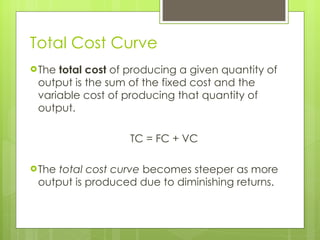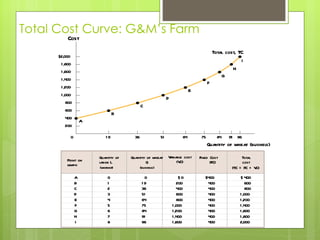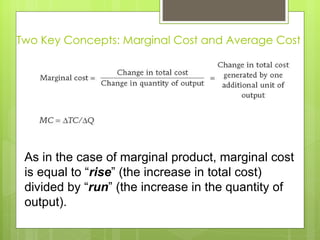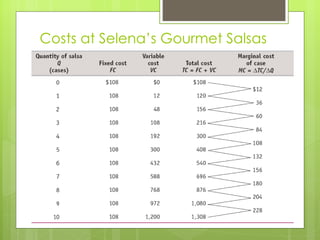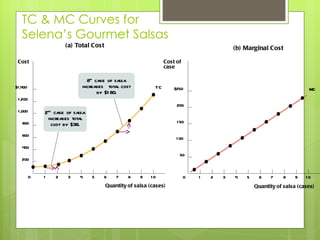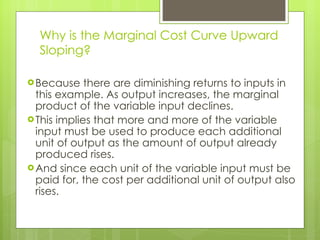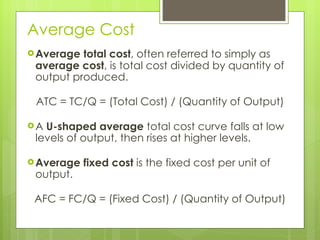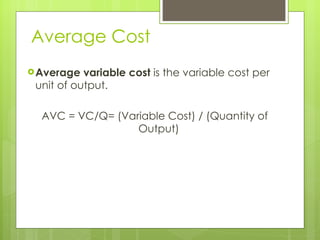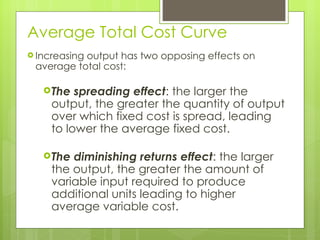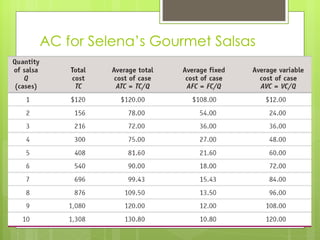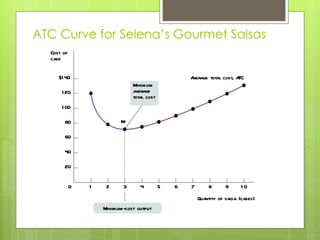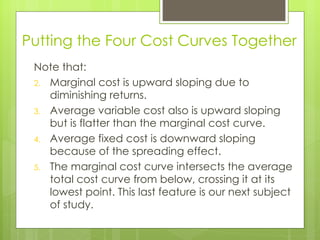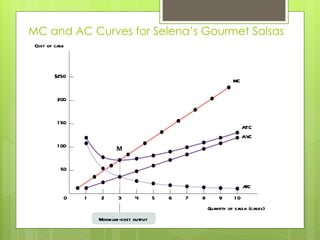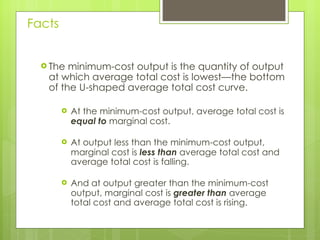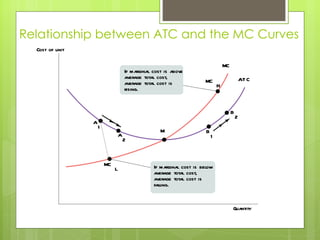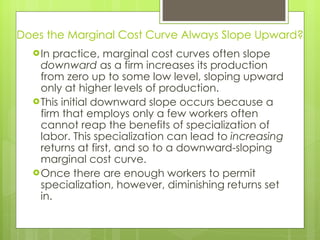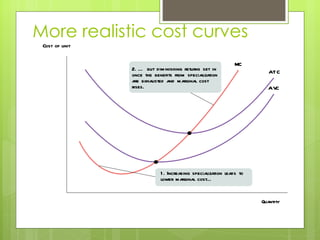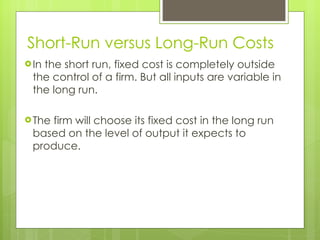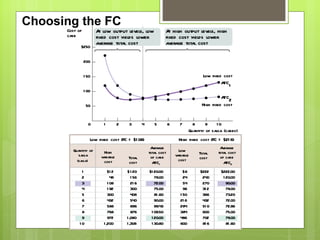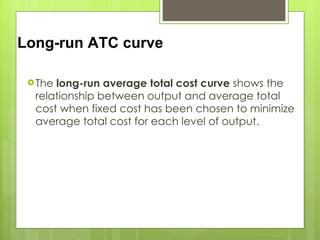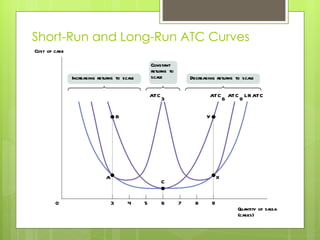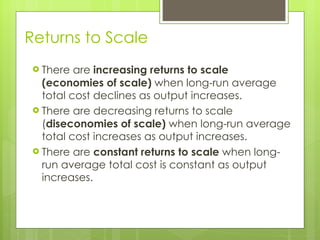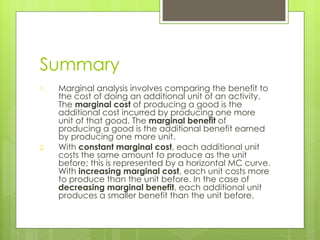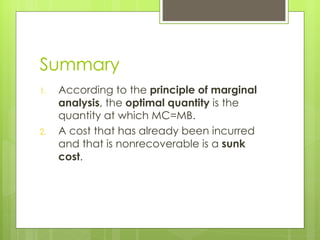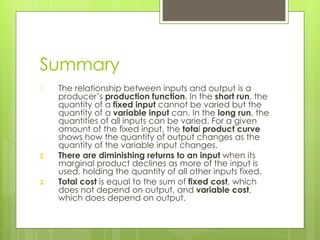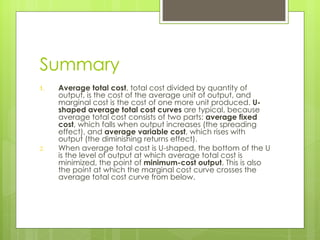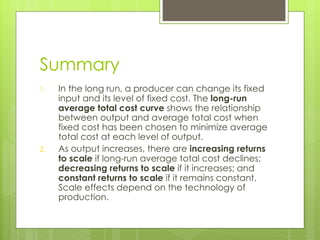1 von 55

### Inputs output costs

• 1. Marginal Analysis, Inputs, Output & Costs [email_address]
• 2.
• 3.
• 4. Opportunity Cost of an Additional Year of School
• 5.
• 6.
• 7. Profits at B ’s Cafe
• 8. Marginal Cost The marginal cost of producing a good is the additional cost incurred by producing one more unit of that good.
• 9.
• 10. Marginal Cost 7 6 5 4 3 2 1 0 \$4.00 3.00 2.00 1.00 Marginal cost (per wing) Quantity of chicken wings Marginal cost curve, MC
• 11.
• 12.
• 13.
• 14. Marginal Benefit 7 6 5 4 3 2 1 0 Marginal benefit curve, MB Marginal cost (per wing) Quantity of chicken wings
• 15. Felix ’s Net Gain from Mowing Lawns
• 16.
• 17. B ’s net gain from increasing portion size
• 18. B ’s optimal portion size 7 6 5 4 3 2 1 0 \$4.00 3.00 2.00 1.00 Marginal benefit (marginal cost per wing) Quantity of chicken wings Optimal point MC MB Optimal quantity 0 1 2 3 4 5 6 7 Total net gain (profit per serving) Net gain (per wing) Quantity of chicken wings \$0 3.50 5.20 5.90 6.30 6.40 6.30 6.10 \$3.50 1.70 0.70 0.40 0.10 – 0.10 – 0.20
• 19.
• 20.
• 21.
• 22. Production function (total output curve) for a farm 0 1 2 3 4 5 6 7 8 19 17 15 13 11 9 7 5 0 19 36 51 64 75 84 91 96 Quantity of labor L (worker) Quantity of wheat Q (bushels) MP of labor MPL =  Q /  L (bushels per worker) 7 8 6 5 4 3 2 1 0 100 80 60 40 20 Quantity of wheat (bushels) Quantity of labor (workers) Total product, TP Adding a 7 th worker leads to an increase in output of only 7 bushels Adding a 2 nd worker leads to an increase in output of only 17 bushels
• 23.
• 24.
• 25. Marginal Product of Labor Curve Marginal product of labor, MPL 7 8 6 5 4 3 2 1 0 19 17 15 13 11 9 7 5 Marginal product of labor (bushels per worker) Quantity of labor (workers) There are diminishing returns to labor.
• 26. (a) Total Product Curves (b) Marginal Product Curves Marginal product of labor (bushels per worker) Quantity of wheat (bushels) 7 8 6 5 4 3 2 1 0 30 25 20 15 10 5 7 8 6 5 4 3 2 1 0 160 140 120 100 80 60 40 20 TP 20 TP 10 MPL 20 MPL 10 Quantity of labor (workers) Quantity of labor (workers) Total Product, Marginal Product, and the Fixed Input
• 27.
• 28.
• 29. Total Cost Curve: G&M ’s Farm 19 36 51 64 75 84 91 96 0 \$2,000 1,800 1,600 1,400 1,200 1,000 800 600 400 200 Cost Quantity of wheat (bushels) A B C D E F G Total cost, TC H I A B C D E F G H I Point on graph 0 1 2 3 4 5 6 7 8 \$400 400 400 400 400 400 400 400 400 O 200 400 600 800 1,000 1,200 1,400 1,600 400 600 800 1,000 1,200 1,400 1,600 1,800 2,000 0 19 36 51 64 75 84 91 96 Variable cost (VC) Total cost (TC = FC + VC) \$ \$ Quantity of labor L (worker) Quantity of wheat Q (bushels) Fixed Cost (FC)
• 30. Two Key Concepts: Marginal Cost and Average Cost As in the case of marginal product, marginal cost is equal to “ rise ” (the increase in total cost) divided by “ run ” (the increase in the quantity of output).
• 31. Costs at Selena ’s Gourmet Salsas
• 32. TC & MC Curves for Selena ’s Gourmet Salsas \$250 200 150 100 50 Cost of case 7 8 9 10 6 5 4 3 2 1 0 \$1,400 1,200 1,000 800 600 400 200 Cost Quantity of salsa (cases) 7 8 9 10 6 5 4 3 2 1 0 (b) Marginal Cost (a) Total Cost T C MC Quantity of salsa (cases) 8 th case of salsa increases total cost by \$180. 2 nd case of salsa increases total cost by \$36.
• 33.
• 34.
• 35.
• 36.
• 37. AC for Selena ’s Gourmet Salsas
• 38. ATC Curve for Selena ’s Gourmet Salsas Average total cost, ATC M 7 8 9 10 6 5 4 3 2 1 0 \$140 120 100 80 60 40 20 Minimum average total cost Minimum-cost output Cost of case Quantity of salsa (cases)
• 39.
• 40. MC and AC Curves for Selena ’s Gourmet Salsas \$250 200 150 100 50 7 8 9 10 6 5 4 3 2 1 M 0 MC A T C A VC AFC Minimum-cost output Cost of case Quantity of salsa (cases)
• 41.
• 42. Relationship between ATC and the MC Curves Cost of unit Quantity MC A T C MC L MC H A 1 B 1 A 2 B 2 M If marginal cost is above average total cost, average total cost is rising. If marginal cost is below average total cost, average total cost is falling.
• 43.
• 44. More realistic cost curves MC A T C A VC Cost of unit Quantity 2. … but diminishing returns set in once the benefits from specialization are exhausted and marginal cost rises. 1. Increasing specialization leads to lower marginal cost…
• 45.
• 46. Choosing the FC \$250 200 150 100 50 Cost of case Quantity of salsa (cases) 7 8 9 10 6 5 4 3 2 1 0 High fixed cost Low fixed cost A T C 2 A T C 1 At low output levels, low fixed cost yields lower average total cost At high output levels, high fixed cost yields lower average total cost A T C 1 12 48 108 192 300 432 588 768 972 1,200 \$ 120 156 216 300 408 540 696 876 1,080 1,308 Total cost \$ A T C 2 6 24 54 96 150 216 294 384 486 600 \$ \$222 240 270 312 366 432 510 600 702 816 Low fixed cost (FC = \$108) High fixed cost (FC = \$216) \$120.00 78.00 72.00 75.00 81.60 90.00 99.43 109.50 120.00 130.80 \$222.00 120.00 90.00 78.00 73.20 72.00 72.86 75.00 78.00 81.60 1 2 3 4 5 6 7 8 9 10 Average total cost of case Quantity of salsa (salsa) High variable cost Low variable cost Total cost Average total cost of case
• 47.
• 48. Short-Run and Long-Run ATC Curves B A T C 6 A T C 9 A T C 3 L R A T C 3 5 8 4 7 0 6 9 Increasing returns to scale Decreasing returns to scale Constant returns to scale C X A Y Cost of case Quantity of salsa (cases)
• 49.
• 50.
• 51.
• 52.
• 53.
• 54.
• 55.

### Hinweis der Redaktion

1. Figure Caption: Figure 9-1: Marginal Cost The height of each shaded bar corresponds to Babette ’s marginal cost of producing an additional chicken wing per serving. The height of each bar here is the same, \$0.80, because Babette has constant marginal cost. So Babette’s marginal cost curve, the line connecting the midpoints at the top of each bar, is horizontal.
2. Figure Caption: Figure 9-2: Marginal Benefit The height of each shaded bar corresponds to Babette ’s marginal benefit of producing an additional chicken wing per serving. The height of each bar is lower than the one preceding it because Babette has decreasing marginal benefit. That is, the additional amount that Babette’s customers are willing to pay for an additional chicken wing falls as the portion size gets larger and larger. As a result, Babette’s marginal benefit curve, the curve connecting the midpoints at the top of each bar, slopes downward.
3. Figure Caption: Figure 9-3: Babette ’s Optimal Portion Size The optimal quantity is the quantity that generates the highest possible total net gain. It is the quantity at which marginal benefit is equal to marginal cost. Equivalently, it is the quantity at which marginal benefit and marginal cost curves intersect. Here, they intersect at a portion size of approximately 5 wings. The table confirms that 5 is indeed the optimal quantity: it leads to the maximum total net gain, or profit, of \$6.40.
4. Figure Caption: Figure 12-1: Production Function and Total Product Curve for George and Martha ’s Farm The table shows the production function, the relationship between the quantity of the variable input (labor, measured in number of workers) and the quantity of output (wheat, measured in bushels) for a given quantity of the fixed input. It also calculates the marginal product of labor on George and Martha ’s farm. The total product curve shows the production function graphically. It slopes upward because more wheat is produced as more workers are employed. It also becomes flatter because the marginal product of labor declines as more and more workers are employed.
5. Figure Caption: Figure 12-2: Marginal Product of Labor Curve for George and Martha ’s Farm The marginal product of labor curve plots each worker ’s marginal product, the increase in the quantity of output generated by each additional worker. The change in the quantity of output is measured on the vertical axis and the number of workers employed on the horizontal axis. The first worker employed generates an increase in output of 19 bushels, the second worker generates an increase of 17 bushels, and so on. The curve slopes downward due to diminishing returns to labor.
6. Figure Caption: Figure 12-3: Total Product, Marginal Product, and the Fixed Input This figure shows how the quantity of output—illustrated by the total product curve—and marginal product depend on the level of the fixed input. Panel (a) shows two total product curves for George and Martha ’s farm, TP10when their farm is 10 acres and TP20when it is 20 acres. With more land, each worker can produce more wheat. So an increase in the fixed input shifts the total product curve up from TP10to TP20. This also implies that the marginal product of each worker is higher when the farm is 20 acres than when it is 10 acres. As a result, an increase in acreage also shifts the marginal product of labor curve up from MPL 10 to MPL 20 . Panel (b) shows the marginal product of labor curves. Note that both marginal product of labor curves still slope downward due to diminishing returns to labor.
7. Figure Caption: Figure 12-4: Total Cost Curve for George and Martha ’s Farm The table shows the variable cost, fixed cost, and total cost for various output quantities on George and Martha ’s 10-acre farm. The total cost curve shows how total cost (measured on the vertical axis) depends on the quantity of output (measured on the horizontal axis). The labeled points on the curve correspond to the rows of the table. The total cost curve slopes upward because the number of workers employed, and hence total cost, increases as the quantity of output increases. The curve gets steeper as output increases due to diminishing returns to labor.
8. Figure Caption: Figure 12-6: Total Cost and Marginal Cost Curves for Selena ’s Gourmet Salsas Panel (a) shows the total cost curve from Table 12-1. Like the total cost curve in Figure 12-4, it slopes upward and gets steeper as we move up it to the right. Panel (b) shows the marginal cost curve. It also slopes upward, reflecting diminishing returns to the variable input.
9. Figure Caption: Figure 12-7: Average Total Cost Curve for Selena ’s Gourmet Salsas The average total cost curve at Selena ’s Gourmet Salsas is U-shaped. At low levels of output, average total cost falls because the “spreading effect” of falling average fixed cost dominates the “diminishing returns effect” of rising average variable cost. At higher levels of output, the opposite is true and average total cost rises. At point M, corresponding to an output of three cases of salsa per day, average total cost is at its minimum level, the minimum average total cost.
10. Figure Caption: Figure 12-8: Marginal Cost and Average Cost Curves for Selena ’s Gourmet Salsas Here we have the family of cost curves for Selena ’s Gourmet Salsas: the marginal cost curve (MC), the average total cost curve (ATC), the average variable cost curve (AVC), and the average fixed cost curve (AFC). Note that the average total cost curve is U-shaped and the marginal cost curve crosses the average total cost curve at the bottom of the U, point M, corresponding to the mini- mum average total cost from Table 12-2 and Figure 12-7.
11. Figure Caption: Figure 12-9: The Relationship Between the Average Total Cost and the Marginal Cost Curves To see why the marginal cost curve (MC) must cut through the average total cost curve at the minimum average total cost (point M), corresponding to the minimum-cost output, we look at what happens if marginal cost is different from average total cost. If marginal cost is less than average total cost, an increase in output must reduce average total cost, as in the movement from A1to A2. If marginal cost is greater than average total cost, an increase in output must increase average total cost, as in the movement from B1 to B2.
12. Figure Caption: Figure 12-10: More Realistic Cost Curves A realistic marginal cost curve has a “swoosh” shape. Starting from a very low output level, marginal cost often falls as the firm increases output. That’s because hiring additional workers allows greater specialization of their tasks and leads to increasing returns. Once specialization is achieved, however, diminishing returns to additional workers set in and marginal cost rises. The corresponding average variable cost curve is now U-shaped, like the average total cost curve. Marginal cost curves do not always slope upward. The benefits of specialization of labor can lead to increasing returns at first represented by a downward-sloping marginal cost curve. Once there are enough workers to permit specialization, however, diminishing returns set in.
13. Figure Caption: Figure 12-11: Choosing the Level of Fixed Cost for Selena ’s Gourmet Salsas There is a trade-off between higher fixed cost and lower variable cost for any given output level, and vice versa. ATC 1 is the average total cost curve corresponding to a fixed cost of \$108; it leads to lower fixed cost and higher variable cost. ATC 2 is the average total cost curve corresponding to a higher fixed cost of \$216 but lower variable cost. At low output levels, at 4 or fewer cases of salsa per day, ATC 1 lies below ATC 2 : average total cost is lower with only \$108 in fixed cost. But as output goes up, average total cost is lower with the higher amount of fixed cost, \$216: at more than 4 cases of salsa per day, ATC 2 lies below ATC 1 .
14. Figure Caption: Figure 12-12: Short-Run and Long-Run Average Total Cost Curves Short-run and long-run average total cost curves differ because a firm can choose its fixed cost in the long run. If Selena has chosen the level of fixed cost that minimizes short-run average total cost at an output of 6 cases, and actually produces 6 cases, then she will be at point Con LRATC and ATC 6 . But if she produces only 3 cases, she will move to point B. If she expects to produce only 3 cases for a long time, in the long run she will reduce her fixed cost and move to point A on ATC 3 . Likewise, if she produces 9 cases (putting her at point Y) and expects to continue this for a long time, she will increase her fixed cost in the long run and move to point X.